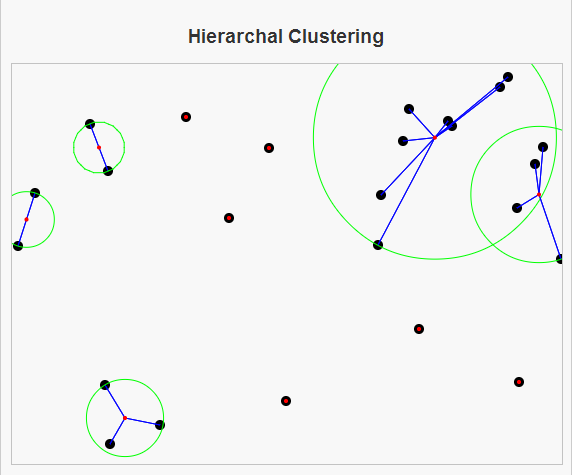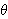# Hierarchical ClusteringHierarchical Clustering algorithms give a nice introduction for computer science students to unsupervised machine learning. I say this because the bottom-up approach to Hierarchical clustering (which I have implemented here) is very similar to Kruskal’s algorithm for finding the minimum spanning tree of a graph.

In Kruskal’s algorithm, we begin by creating a forest, or a set of trees where each node is its own tree. The algorithm then selects the two trees that are closest together (closest being defined as the minimum cost edge between two distinct trees) and merges those trees together. This process of merging the closest two trees is then repeated until there is only one tree remaining, which is a minimum spanning tree of the graph.

Similarly, bottom-up hierarchical clustering of a group of points begins by saying that each point is its own cluster. Then the clusters are compared to one another to check if two clusters will be merged into one. Generally, there will be some stopping criteria,, saying that we do not want to merge two clusters together if their distance is greater than. So if the minimum distance between two clusters is less thanwe will proceed as in Kruskal’s algorithm by merging these two clusters together into one cluster. We repeat this process of merging the closest two clusters together until we find that the minimum distance between two clusters is greater than or equal to, in which case we can stop and the result is a partition of our data set into distinct clusters.

Hierarchical clustering is comparable to K-Means Clustering. Here are some differences between the two approaches:

1. K-Means Clustering requires an initial number of desired clusters, while Hierarchical clustering does not.
2. A run of K-Means Clustering will always give K clusters, whereas Hierarchical Clustering can give more or less, depending on our tolerance.
3. K-Means can undo previous mistakes (assignments of an element to the wrong cluster), while Hierarchical Clustering cannot.

So, here is a link to my page on Hierarchical Clustering. Hope you enjoy.